## Force System with Permanent Magnets:

Method of finding force system with permanent magnets is best illustrated by an example. Figure 4.17(b) shows a moving armature relay excited by a permanent magnet (PM).

The dc magnetizing curve of the permanent magnet is drawn in Fig. 4.17(a) which upon linear extrapolation at the lower B-end can be expressed as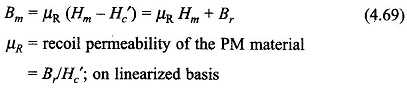Soft iron portion of the magnetic circuit including the armature is assumed to have μ = ∞. For finding the force on the armature, it will be convenient to use Eq. (4.25) for which we will need the expression of system co-energy W′f (i, x) which must be independent of i as there is no exciting current in the system. Thus coenergy will be a function of the space variable x only i.e.,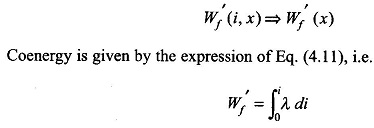This expression needs to be carefully interpreted for the case of permanent magnetic excited systems. The limits of integration in this expression mean from state of zero flux to a state of certain flux (but with zero current). The state of zero flux is imagined by means of a fictitious exciting coil (of Nf turns) carrying current if as shown in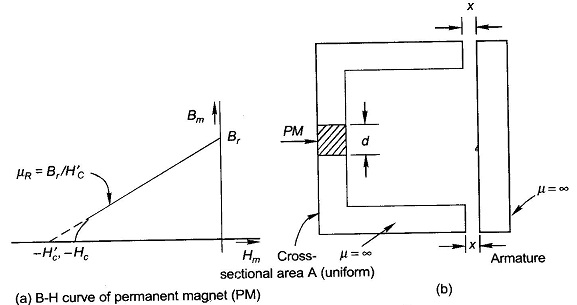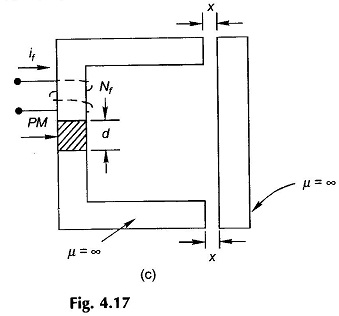Fig. 4.17(c). The current is assumed to be adjusted to value If causing the core flux to reduce to zero and the original state is then reached by imaging the current (if) to reduce to zero. ThusThe force on the armature is then given byLet us now calculate the magnitude of the force for typical numerical values as below,Note: Negative sign in force is indicative of the fact that the force acts in a direction to reduce x (air-gap).

Similar treatment could be used for mixed situation where system has both permanent magnets and exciting coils. It should be stressed here that an alternative procedure is to use the finite element method to evaluate the coenergy from the vector form of Eq. (4.18a) integrated over the volume, i.e.,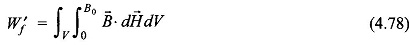To calculate force -dW′f/dx is obtained by numerical differentiation. This method is of general applicability wherever the magnetic circuit analysis cannot be carried out.